# Implement Hardware-Efficient Real Burst Matrix Solve Using Q-less QR Decomposition with Forgetting Factor

This example shows how to use the hardware-efficient Real Burst Matrix Solve Using Q-less QR Decomposition with Forgetting Factor block.

### Q-less QR Decomposition with Forgetting Factor

The Real Burst Matrix Solve Using Q-less QR Decomposition with Forgetting Factor block implements the following recursion to compute the upper-triangular factor R of continuously streaming n-by-1 row vectors A(k,:) using forgetting factor. It's as if matrix A is infinitely tall. The forgetting factor in the rangekeeps it from integrating without bound.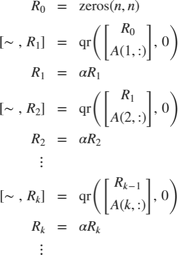### Forward and Backward Substitution

When an upper triangular factor is ready, then forward and backward substitution are computed with the current input B to produce output X.### AMBA AXI Handshaking Process

The Data Handler subsystem in this model takes real matrices A and B as inputs. It sends rows of A and full matrix of B to QR Decomposition block using the AMBA AXI handshake protocol. The validIn signal indicates when data is available. The ready signal indicates that the block can accept the data. Transfer of data occurs only when both the validIn and ready signals are high. You can set delays for the feeding in rows of A and the feeding in B matrices in the Data Handler to emulate the processing time of the upstream block. validInA and validInB remain high when aDelay and bDelay are set to 0 because this indicates the Data Handler always has data available.

### Asynchronous Matrix Solver

This block operates asynchronously. First, Q-less QR decomposition is performed on the input A matrix and the resulting R matrix is put into a buffer. Then, the Forward Backward Substitute block uses the input B matrix and the buffered R matrix to compute R'RX = B. Because the R and B matrices are stored separately in buffers, the upstream Q-less QR decomposition block and the downstream Forward Backward Substitute block can run independently. The Forward Backward Substitute block starts processing when the first R and B matrices are available. Then it runs continuously using the latest buffered R and B matrices, regardless of the status of the Q-less QR Decomposition block. For example, if the upstream block stops providing A and B matrices, the Forward Backward Substitute block continues to generate the same output using the last pair of R and B matrices.

### Define System Parameters

n is the length of the row vectors A(k,:), the number of rows in B, and the number of rows and columns in R.

n = 5; 

p is the number of columns in B

p = 1; 

m is the effective numbers of rows of A to integrate over.

m = 100; 

Use the fixed.forgettingFactor function to compute the forgetting factor as a function of the number of rows that you are integrating over.

forgettingFactor = fixed.forgettingFactor(m) 
forgettingFactor = 0.9950 

precisionBits defines the number of bits of precision required for the QR Decomposition. Set this value according to system requirements.

precisionBits = 24; 

In this example, real-valued matrices A and B are constructed such that the magnitude of their real and imaginary parts of their elements is less than or equal to one, so the maximum possible absolute value of any element is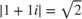. Your own system requirements will define what those values are. If you don't know what they are, and A and B are fixed-point input to the system, then you can use the upperbound function to determine the upper bounds of the fixed-point types of A and B.

max_abs_A is an upper bound on the maximum magnitude element of A.

max_abs_A = sqrt(2); 

max_abs_B is an upper bound on the maximum magnitude element of B.

max_abs_B = sqrt(2); 

### Select Fixed-Point Types

Use the fixed.realQlessQRMatrixSolveFixedpointTypes function to compute fixed-point types.

T = fixed.realQlessQRMatrixSolveFixedpointTypes(m,n,max_abs_A,max_abs_B,precisionBits); 

T.A is the fixed-point type computed for transforming A to R in-place so that it does not overflow.

T.A 
ans = [] DataTypeMode: Fixed-point: binary point scaling Signedness: Signed WordLength: 31 FractionLength: 24 

T.B is the type computed for B so that it does not overflow.

T.B 
ans = [] DataTypeMode: Fixed-point: binary point scaling Signedness: Signed WordLength: 27 FractionLength: 24 

T.X is the type computed for the output X so that there is a low probability of overflow.

T.X 
ans = [] DataTypeMode: Fixed-point: binary point scaling Signedness: Signed WordLength: 77 FractionLength: 24 

### Define Simulation Parameters

Create random matrix A to contain a specified number of inputs, and n-by-p random matrix B.

numInputs is the number of input rows A(k,:) for this example.

numInputs = 500; rng('default') [A,B] = fixed.example.realRandomQlessQRMatrices(numInputs,n,p); 

Cast the inputs to the types determined by fixed.complexQlessQRMatrixSolveFixedpointTypes.

A = cast(A,'like',T.A); B = cast(B,'like',T.B); 

Use the fixed.extractNumericType function to extract a numerictype object to use as an input parameter to the block.

OutputType = fixed.extractNumericType(T.X) 
OutputType = DataTypeMode: Fixed-point: binary point scaling Signedness: Signed WordLength: 77 FractionLength: 24 

Cast the forgetting factor to a fixed-point type with the same word length as A and best-precision scaling.

forgettingFactor = fi(forgettingFactor,1,T.A.WordLength); 

Set delay for feeding in rows of A

aDelay = 1; 

Set delay for feeding in B matrices

bDelay = 1; 

Select a stop time for the simulation that is long enough to process all the inputs from A.

stopTime = 2*(2*numInputs + n)*T.A.WordLength; 

### Open the Model

model = 'RealBurstSolveQlessQRForgettingFactorModel'; open_system(model);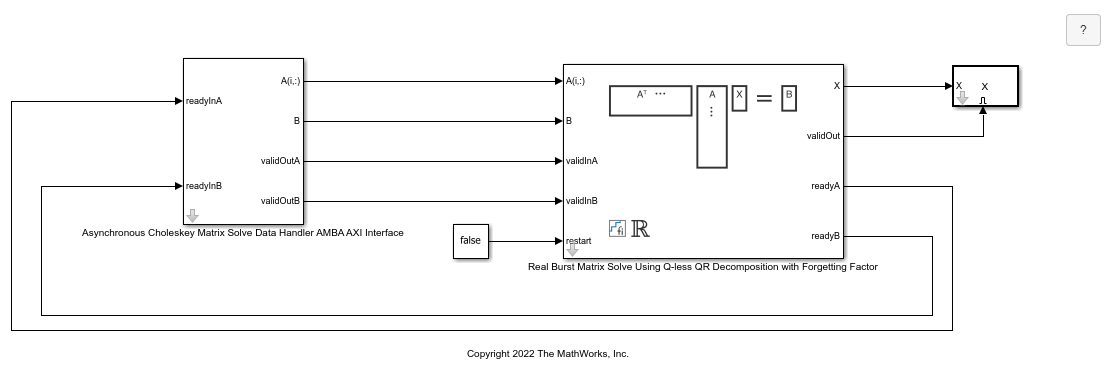### Set Variables in the Model Workspace

Use the helper function setModelWorkspace to add the variables defined above to the model workspace.

fixed.example.setModelWorkspace(model,'A',A,'B',B,'n',n,'p',p,... 'forgettingFactor',forgettingFactor,'OutputType',OutputType,... 'regularizationParameter',0,... 'aDelay',aDelay,'bDelay',bDelay,... 'stopTime',stopTime); 

### Simulate the Model

out = sim(model); 

### Verify the Accuracy of the Output

Define matrix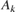as followsThen using the formula for the computation of theth output, and the fact that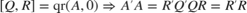, you can show thatSo to verify the output, the difference betweenand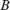should be small.

Choose the last output of the simulation.

X = double(out.X(:,:,end)); 

Synchronize the last output X with the input by finding the number of inputs that produced it.

A = double(A); B = double(B); alpha = double(forgettingFactor); relative_errors = nan(1,numInputs); for k = 1:numInputs A_k = alpha.^(k:-1:1)' .* A(1:k,:); relative_errors(k) = norm(A_k'*A_k*X - B)/norm(B); end 

k is the number of inputs A(k,:) that produced the last X.

k = find(relative_errors==min(relative_errors)) 
k = 329 

Verify that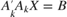with a small relative error.

A_k = alpha.^(k:-1:1)' .* A(1:k,:); relative_error = norm(A_k'*A_k*X - B)/norm(B) 
relative_error = 7.6410e-04 

Suppress mlint warnings in this file.

%#ok<*NOPTS>